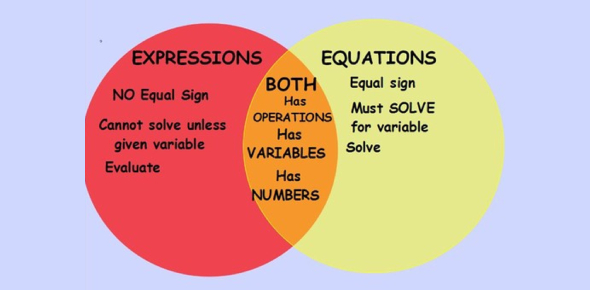# Ultimate Math Test On Expressions And Equations! Trivia Quiz

26 Questions | Attempts: 994
ShareSettings.

• 1.
The expression "four less than a number" is the same as which of the following?
• A.

4-x

• B.

X-4

• 2.
Brett got on an elevator and rode up 10 floors.  Next he rode down 7 floors.  After that he went up 4 floors.  Finally he went down 6 floors.  When he got off the elevator he was on the 11th floor.  What floor was he on when he started his ride?
• A.

8th floor

• B.

9th floor

• C.

10th floor

• D.

12th floor

• 3.
Sara and Kim together have  15 pencils.  Sara has 9  pencils. Mark and Kim together have 10 pencils. How many pencils does Mark have?
• A.

5

• B.

34

• C.

8

• D.

4

• 4.
Steve has x dollars in his bank account.  He deposits \$10 each week for 3 weeks.  His bank balance now is \$70.  What is x? ( How much money did he have to start?)
• A.

\$60

• B.

\$20

• C.

\$40

• D.

\$50

• 5.
What is the algebraic expression for "a number f decreased by 18"?
• A.

18f

• B.

F + 18

• C.

F - 18

• D.

18 + f

• 6.
If m = 17, what is the sum of 5 and m?
• A.

85

• B.

22

• C.

21

• D.

84

• 7.
What is the difference between an expression and an equation?
• A.

They are the same thing.

• B.

An equation has an equal sign, but an expression does not.

• C.

An equation has more numbers and an expression has more variables.

• D.

An expression always has a higher value for the variable.

• 8.
Which of the following does NOT mean multiplication?
• A.

Product

• B.

Twice something

• C.

Times

• D.

More than

• 9.
The expression "twice the product of 3 and x" is the same as which of the following?
• A.

2+(3x)

• B.

(2+3)x

• C.

2(3x)

• D.

2(3+x)

• 10.
Which of the following is the expression in words for 3x - 4.
• A.

4 less than the product of 3 and x

• B.

4 less than the quotient of 3 and x

• C.

The product of 3 and x increased by 4

• 11.
What is the algebraic expression for....four times the sum of nine and a number
• A.

4(9 + n)

• B.

4 x 9 + n

• C.

4n + 9

• 12.
Three times Cassie's age increased by nine is twelve.
• A.

3C+12 - 9

• B.

3C + 9=12

• C.

3C + 12 = 9

• 13.
What does 25k mean?
• A.

• B.

The difference between k and 25

• C.

The product of 25 and k

• 14.
Twenty-two times h plus three
• A.

H x 22 + 3

• B.

3 + 2 x 22

• C.

22 x a + 3

• 15.
Twenty-two times a number, a, decreased by three
• A.

22 x a - 3

• B.

3 - a x 22

• C.

22 + a x 3

• 16.
The sum of three and twenty-two, then divided by the number a
• A.

(3 + 22) / a

• B.

A / (3 + 22)

• C.

3 + 22 / a

• 17.
Fill in the blank! A _________ is a letter that represents a number(of something).
• 18.
Please fill answer belowThe ____________ of a variable is the number in front of the variable.
• 19.
The coefficient of x when you have 1.55x is.....
• A.

1.00

• B.

0.55

• C.

1.55

• D.

155

• 20.
The coefficient of y when you have 22y/9 is.....
• A.

1/9

• B.

22/9

• C.

22

• 21.
Translate from an English expression into an Algebraic expression: a decreased by 17 ***answer with NO SPACES***  Example: x+5
• 22.
Translate from an English expression into an Algebraic expression:the ratio of m and n***answer with NO SPACES***  Example: x+5
• 23.
Translate from an English expression into an Algebraic expression:8 less than the sum of a and b***answer with NO SPACES***  Example: x+5
• 24.
Translate from an English expression into an Algebraic expression:15 more than one third x***answer with NO SPACES***  Example: (1/2)x+5***do not use a decimal number for "one third"
• 25.
Translate from an English expression into an Algebraic expression:the quotient of 55 and z***answer with NO SPACES***  Example: x+5

## Related TopicsBack to top
×

Wait!
Here's an interesting quiz for you.